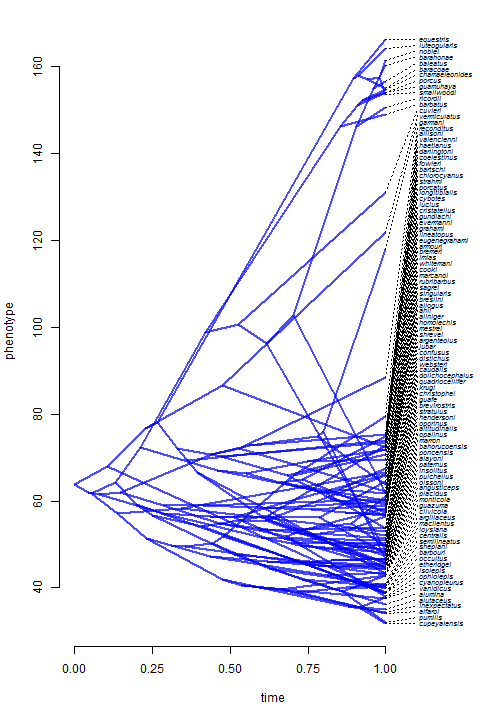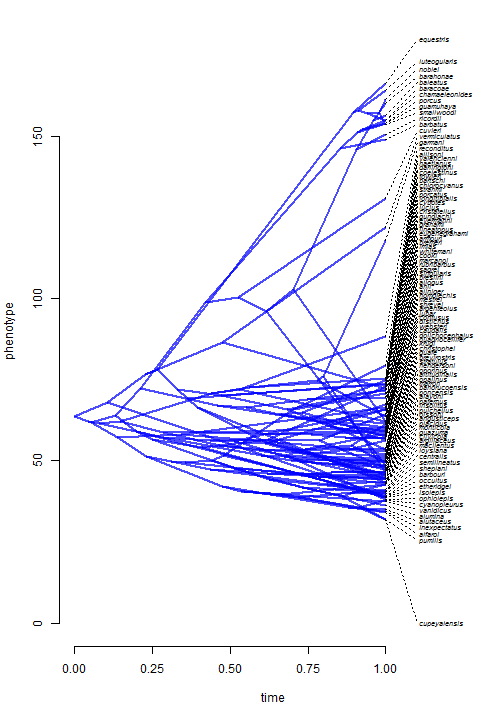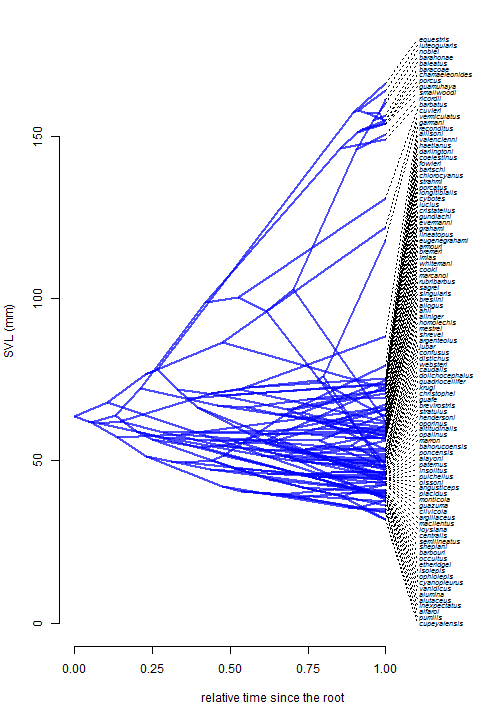## Tuesday, September 19, 2017

### Custom tip label spacing in `phenogram`

“I would like to construct a phenogram for a paper using the phytools (v. 0.6-20) function `phenogram` when `spread.labels` argument is `TRUE` and `ylim` is greater than default range estimated by the function. I would like to provide more space along y axis since I want to 1) incorporate additional figures to the traitgram and 2) to make more space for tips so they are not overlapped. I was able to extend `ylim`, but current function do not take advantage of more space provided by the arbitrary range of `ylim`. Therefore, tips are (still) overlapped making it harder to read. Please, can you tell me if there is a hack that will enable function to exploit the full extent of arbitrary set `ylim`?.”

In fact, this is kind of already possible using the argument `spread.range`. Unfortunately, even this has some limitations.

First, the default:

``````library(phytools)
row.names=1))[,1])
par(mar=c(5.1,4.1,1.1,1.1))
col=make.transparent("blue",0.7))
``````
``````## Optimizing the positions of the tip labels...
``````Now, using `spread.range`:

``````par(mar=c(5.1,4.1,1.1,1.1))
col=make.transparent("blue",0.7),ylim=c(0,180),
``````
``````## Optimizing the positions of the tip labels...
``````What has happened is that although the labels are spread out to utilize more space, the optimization routine does not allow all the labels to be spread optimally, which is unfortunate (in spite of a 0 spread cost to vertical displacement). And, since the label positions are numerically optimized, sometimes weird things even happen - like an errant tip label at one end or the other!

To address this, I today pushed a small update to `phenogram` which allows custom tip label spacing. I'm going to download the source from GitHub, but this function version can also be obtained by simply updating phytools from GitHub using devtools:

``````source("https://raw.githubusercontent.com/liamrevell/phytools/4407c72a4f5076db3bdd6f6eca557ba9ef454045/R/phenogram.R")
labels<-setNames(seq(0,180,by=180/(Ntip(tree)-1)),names(sort(svl)))
par(mar=c(5.1,4.1,1.1,1.1))
phenogram(tree,svl,fsize=0.6,label.pos=sample(labels),
ylim=c(0,180),ftype="i",col=make.transparent("blue",0.7),
xlab="relative time since the root",
ylab="SVL (mm)")
``````
``````## Optimizing the positions of the tip labels...
``````What I did here is just supply evenly spaced vertical positions on the desired range in the order of the trait vector - in this case `svl`.

Neat.